# Epidemics:ferguson2008

## Cauchemez et al Model

An example of so called SIR (Susceptible, Infectious, Recovered) model.

Joint probability of observed (Y), unobserved variables (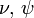$\nu,\,\psi$), and parameters (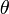$\theta$) is given by: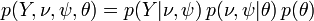$p\big(Y,\nu,\psi,\theta) = p(Y|\nu,\psi)\,p(\nu,\psi|\theta)\,p(\theta)$,

where: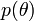$p\big(\theta\big)$,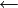$\leftarrow$ prior level (prior distributions of the parameters of the model).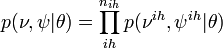$p(\nu,\psi|\theta)=\prod_{ih}^{n_{ih}}p(\nu^{ih},\psi^{ih}|\theta)$,$\leftarrow$ transmission level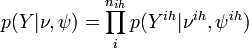$p(Y|\nu,\psi)=\prod_{i}^{n_{ih}}p(Y^{ih}|\nu^{ih},\psi^{ih})$,$\leftarrow$ observation level

Y - indicator function: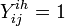$Y_{ij}^{ih}=1$ (for ith, ($i=1,2,..,n_{ih}$) individual of ihth household (of size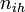$n_{ih}$) on jth day (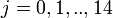$j=0,1,..,14$)), if clinical influenza was observed,$Y_{ij}^{ih}=0$ otherwise.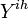$Y^{ih}$ - all observations from household ihth, Y - observations from all households.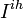$I^{ih}$ - group of individuals at ihth household with at least 1 day of clinical influenza,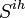$S^{ih}$ - remaining members of the ihth household.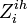$Z_{i}^{ih}$ - the 1st day of clinical influenza of ith individual in ihth household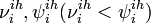$\nu_{i}^{ih},\psi_{i}^{ih} (\nu_{i}^{ih}<\psi_{i}^{ih})$ - unobserved variables corresponding to the start and the end of the infectious period for ith individual of ihth household

### Observation level - parameters$\nu,\,\psi$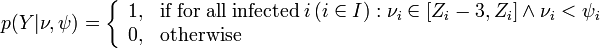$p(Y|\nu,\psi)=\left\{\begin{array}{ll} 1, & \textrm{if\;for\;all\;infected\;}i\,(i\in I): \nu_i\in [Z_i-3,Z_i] \land \nu_i < \psi_i\\ 0, & \textrm{otherwise} \end{array} \right.$

This level ensured that the unobserved data,$\nu,\,\psi$, agreed with observed data, Y.

### Transmission level - parameters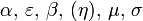$\alpha,\,\varepsilon,\,\beta,\,(\eta),\,\mu,\,\sigma$

The instantaneous risk of infection for an individual at time t in household of size n: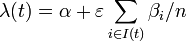$\lambda(t) = \alpha + \varepsilon \sum_{i\in I(t)} \beta_i / n$,

where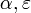$\alpha, \varepsilon$ - instantaneous risk of infection from the community and within household, respectively.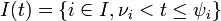$I(t)=\{i\in I,\nu_i - the group of infectives just before time t.

The duration of infectious period for ith infective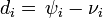$d_i=\,\psi_i-\nu_i$ is taken from the gamma distribution with mean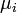$\mu_i$ and standard deviation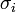$\sigma_i$.
With the above, conditional on the date of the first infection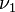$\nu_1$, we have (for the household):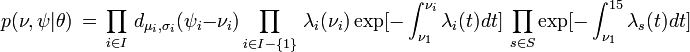$p(\nu,\psi|\theta)\,=\,\prod_{i\in I}\,d_{\mu_i,\sigma_i}(\psi_i-\nu_i)\prod_{i\in I-\{1\}}\,\lambda_i(\nu_i)\exp[-\int_{\nu_1}^{\nu_i}\lambda_i(t)dt]\,\prod_{s\in S}\exp[-\int_{\nu_1}^{15}\lambda_s(t)dt]$
where I-{1} denotes infectives without the first infected

### Prior level

That is prior distributions of all parameters,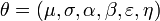$\theta=(\mu, \sigma, \alpha, \beta, \varepsilon, \eta)$

Gamma distribution with mean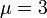$\mu=3$ and standard deviation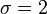$\sigma=2$ was used for the distribution of the duration times of infectious periods. Exponential distribution for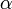$\alpha$ and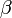$\beta$ were used